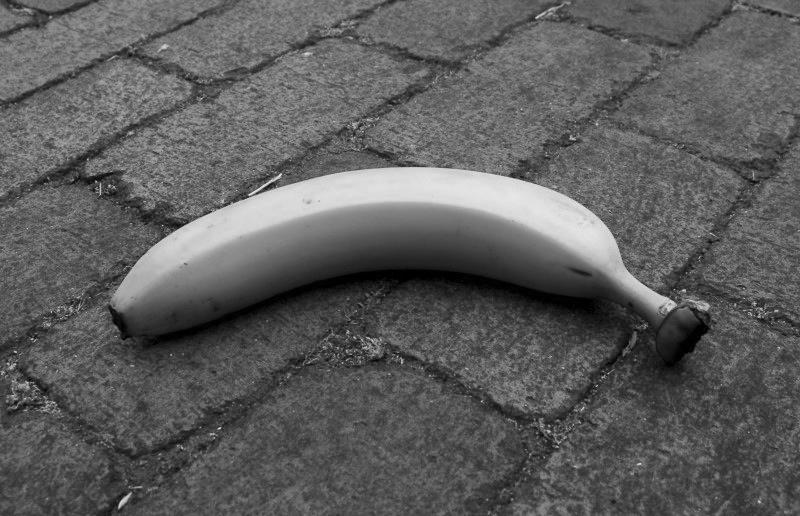You should try and thinking to solve the questions.

View Questions

Although this is a simple question and easy… But it can be interesting for many people.

# Question 1``````pixel.setGreen(pixel.getRed());
pixel.setBlue(pixel.getRed());
``````

# Question 2``````pixel.setGreen(pixel.getRed());
pixel.setBlue(pixel.getRed());
``````

# Question 3``````avg = (pixel.getRed() + pixel.getGreen() + pixel.getBlue())/3;
pixel.setRed(avg);
pixel.setGreen(avg);
pixel.setBlue(avg);
``````

# Question 4``````avg = (pixel.getRed() + pixel.getGreen() + pixel.getBlue())/3;
pixel.setRed(avg);
pixel.setGreen(avg);
pixel.setBlue(avg);
``````